# Smith chart basics

13. Dec 2016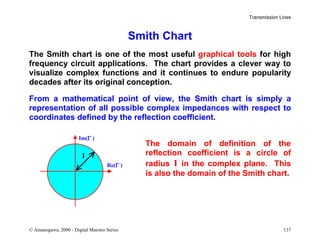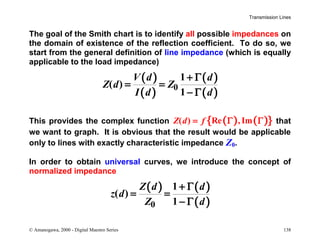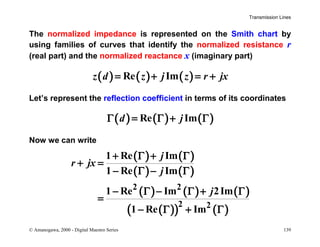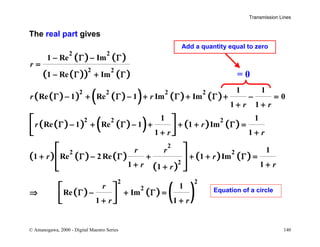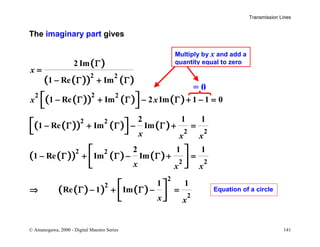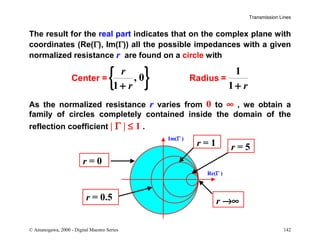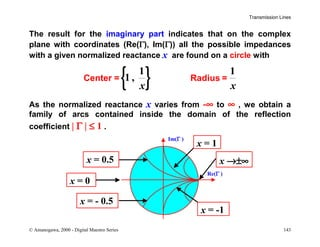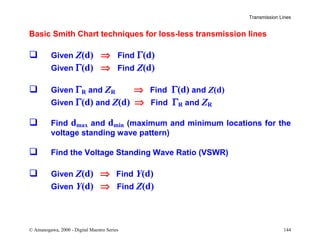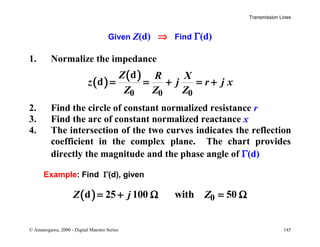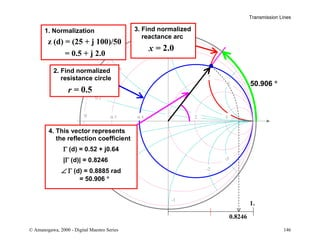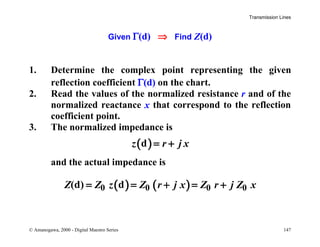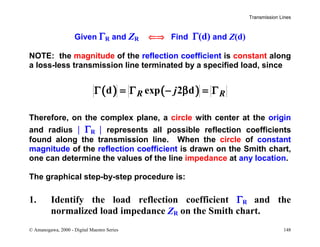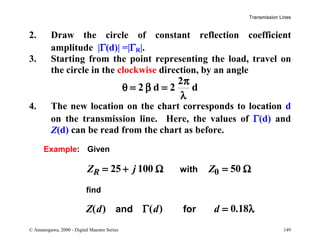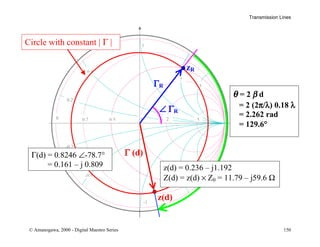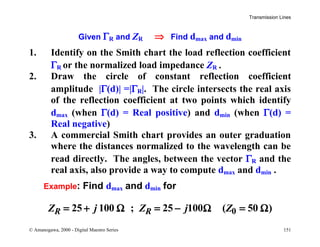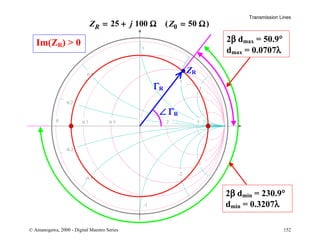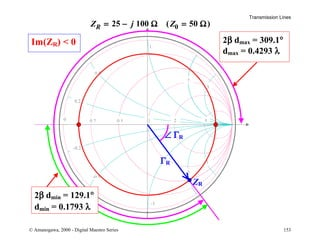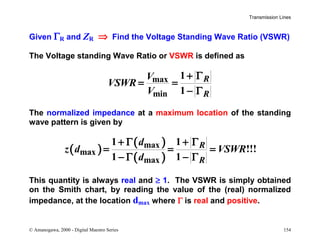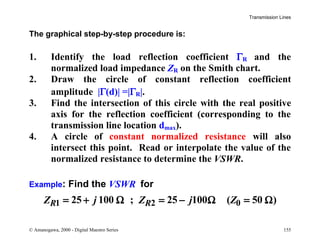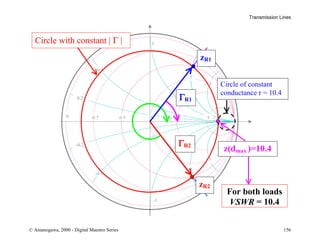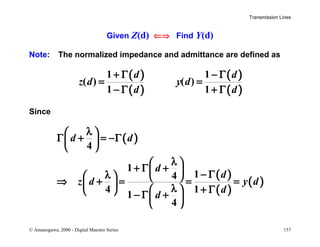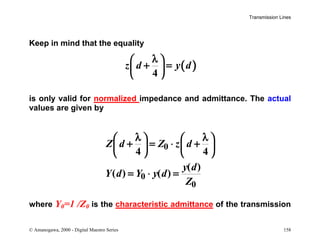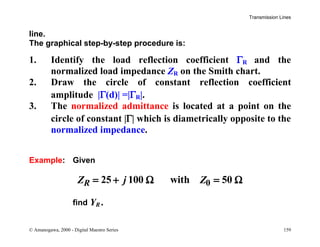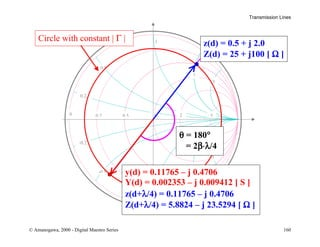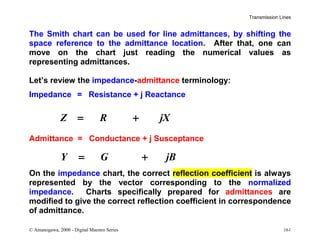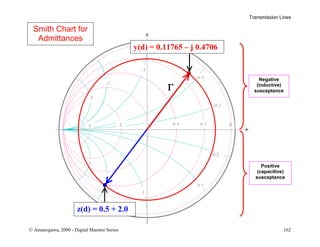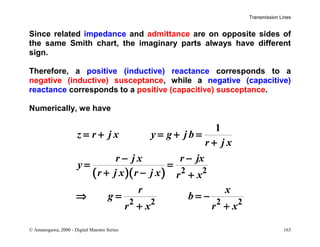1 von 27

### Smith chart basics

• 1. Transmission Lines © Amanogawa, 2000 - Digital Maestro Series 137 Smith Chart The Smith chart is one of the most useful graphical tools for high frequency circuit applications. The chart provides a clever way to visualize complex functions and it continues to endure popularity decades after its original conception. From a mathematical point of view, the Smith chart is simply a representation of all possible complex impedances with respect to coordinates defined by the reflection coefficient. The domain of definition of the reflection coefficient is a circle of radius 1 in the complex plane. This is also the domain of the Smith chart. Im(Γ ) Re(Γ ) 1
• 2. Transmission Lines © Amanogawa, 2000 - Digital Maestro Series 138 The goal of the Smith chart is to identify all possible impedances on the domain of existence of the reflection coefficient. To do so, we start from the general definition of line impedance (which is equally applicable to the load impedance) ( ) ( ) ( ) ( )0 1 ( ) 1 V d d Z d Z I d d + Γ = = − Γ This provides the complex function ( ) ( ){ }( ) Re , ImZ d f= Γ Γ that we want to graph. It is obvious that the result would be applicable only to lines with exactly characteristic impedance Z0. In order to obtain universal curves, we introduce the concept of normalized impedance ( ) ( ) ( )0 1 ( ) 1 Z d d z d Z d + Γ = = − Γ
• 3. Transmission Lines © Amanogawa, 2000 - Digital Maestro Series 139 The normalized impedance is represented on the Smith chart by using families of curves that identify the normalized resistance r (real part) and the normalized reactance x (imaginary part) ( ) ( ) ( )Re Imz d z j z r jx= + = + Let’s represent the reflection coefficient in terms of its coordinates ( ) ( ) ( )Re Imd jΓ = Γ + Γ Now we can write ( ) ( ) ( ) ( ) ( ) ( ) ( ) ( )( ) ( ) 2 2 2 2 1 Re Im 1 Re Im 1 Re Im 2Im 1 Re Im j r jx j j + Γ + Γ + = − Γ − Γ − Γ − Γ + Γ = − Γ + Γ
• 4. Transmission Lines © Amanogawa, 2000 - Digital Maestro Series 140 The real part gives ( ) ( ) ( )( ) ( ) ( )( ) ( )( ) ( ) ( ) ( )( ) ( )( ) ( ) ( ) ( ) ( ) ( ) ( ) ( ) ( ) ( ) ( ) ( ) 2 2 2 2 2 2 2 2 2 2 2 2 2 2 2 2 2 2 1 Re Im 1 Re Im 1 1 Re 1 Re 1 Im Im 0 1 1 1 1 Re 1 Re 1 1 Im 1 1 1 1 Re 2 Re 1 Im 1 11 1 Re Im 1 1 r r r r r r r r r r r r r r rr r r r − Γ − Γ = − Γ + Γ Γ − + Γ − + Γ + Γ + − = + + Γ − + Γ − + + + Γ = + + + Γ − Γ + + + Γ = + ++ ⇒ Γ − + Γ = + +                 = 0 Add a quantity equal to zero Equation of a circle
• 5. Transmission Lines © Amanogawa, 2000 - Digital Maestro Series 141 The imaginary part gives ( ) ( )( ) ( ) ( )( ) ( ) ( ) ( )( ) ( ) ( ) ( )( ) ( ) ( ) ( )( ) ( ) 2 2 22 2 2 2 2 2 2 2 2 2 2 2 2 2 Im 1 Re Im 1 Re Im 2 Im 1 1 0 2 1 1 1 Re Im Im 2 1 1 1 Re Im Im 1 1 Re 1 Im x x x x x x x x x x x Γ = − Γ + Γ − Γ + Γ − Γ + − = − Γ + Γ − Γ + = − Γ + Γ − Γ + = ⇒ Γ − + Γ − =                   = 0 Multiply by x and add a quantity equal to zero Equation of a circle
• 6. Transmission Lines © Amanogawa, 2000 - Digital Maestro Series 142 The result for the real part indicates that on the complex plane with coordinates (Re(Γ), Im(Γ)) all the possible impedances with a given normalized resistance r are found on a circle with { } 1 , 0 1 1 r r r+ + Center = Radius = As the normalized resistance r varies from 0 to ∞ , we obtain a family of circles completely contained inside the domain of the reflection coefficient | Γ | ≤ 1 . Im(Γ ) Re(Γ ) r = 0 r →∞ r = 1 r = 0.5 r = 5
• 7. Transmission Lines © Amanogawa, 2000 - Digital Maestro Series 143 The result for the imaginary part indicates that on the complex plane with coordinates (Re(Γ), Im(Γ)) all the possible impedances with a given normalized reactance x are found on a circle with { }1 1 1 , x x Center = Radius = As the normalized reactance x varies from -∞ to ∞ , we obtain a family of arcs contained inside the domain of the reflection coefficient | Γ | ≤ 1 . Im(Γ ) Re(Γ ) x = 0 x →±∞ x = 1 x = 0.5 x = -1 x = - 0.5
• 8. Transmission Lines © Amanogawa, 2000 - Digital Maestro Series 144 Basic Smith Chart techniques for loss-less transmission lines Given Z(d) ⇒ Find Γ(d) Given Γ(d) ⇒ Find Z(d) Given ΓR and ZR ⇒ Find Γ(d) and Z(d) Given Γ(d) and Z(d) ⇒ Find ΓR and ZR Find dmax and dmin (maximum and minimum locations for the voltage standing wave pattern) Find the Voltage Standing Wave Ratio (VSWR) Given Z(d) ⇒ Find Y(d) Given Y(d) ⇒ Find Z(d)
• 9. Transmission Lines © Amanogawa, 2000 - Digital Maestro Series 145 Given Z(d) ⇒ Find Γ(d) 1. Normalize the impedance ( ) ( ) 0 0 0 d d Z R X z j r j x Z Z Z = = + = + 2. Find the circle of constant normalized resistance r 3. Find the arc of constant normalized reactance x 4. The intersection of the two curves indicates the reflection coefficient in the complex plane. The chart provides directly the magnitude and the phase angle of Γ(d) Example: Find Γ(d), given ( ) 0d 25 100 with 50Z j Z= + Ω = Ω
• 10. Transmission Lines © Amanogawa, 2000 - Digital Maestro Series 146 1 -1 0 0.2 0.5 5 0.2 -0.2 21 -0 5 0 5 -3 3 2 -2 1. Normalization z (d) = (25 + j 100)/50 = 0.5 + j 2.0 2. Find normalized resistance circle r = 0.5 3. Find normalized reactance arc x = 2.0 4. This vector represents the reflection coefficient Γ (d) = 0.52 + j0.64 |Γ (d)| = 0.8246 ∠∠ Γ (d) = 0.8885 rad = 50.906 ° 50.906 ° 1. 0.8246
• 11. Transmission Lines © Amanogawa, 2000 - Digital Maestro Series 147 Given Γ(d) ⇒ Find Z(d) 1. Determine the complex point representing the given reflection coefficient Γ(d) on the chart. 2. Read the values of the normalized resistance r and of the normalized reactance x that correspond to the reflection coefficient point. 3. The normalized impedance is ( )dz r j x= + and the actual impedance is ( ) ( )0 0 0 0(d) dZ Z z Z r j x Z r j Z x= = + = +
• 12. Transmission Lines © Amanogawa, 2000 - Digital Maestro Series 148 Given ΓR and ZR ⇐⇒ Find Γ(d) and Z(d) NOTE: the magnitude of the reflection coefficient is constant along a loss-less transmission line terminated by a specified load, since ( ) ( )d exp 2 dR RjΓ = Γ − β = Γ Therefore, on the complex plane, a circle with center at the origin and radius | ΓR | represents all possible reflection coefficients found along the transmission line. When the circle of constant magnitude of the reflection coefficient is drawn on the Smith chart, one can determine the values of the line impedance at any location. The graphical step-by-step procedure is: 1. Identify the load reflection coefficient ΓR and the normalized load impedance ZR on the Smith chart.
• 13. Transmission Lines © Amanogawa, 2000 - Digital Maestro Series 149 2. Draw the circle of constant reflection coefficient amplitude |Γ(d)| =|ΓR|. 3. Starting from the point representing the load, travel on the circle in the clockwise direction, by an angle 2 2 d 2 d π θ = β = λ 4. The new location on the chart corresponds to location d on the transmission line. Here, the values of Γ(d) and Z(d) can be read from the chart as before. Example: Given 025 100 50RZ j Z= + Ω = Ωwith find ( ) ( ) 0.18Z d d dΓ = λand for
• 14. Transmission Lines © Amanogawa, 2000 - Digital Maestro Series 150 θ 1 -1 0 0.2 0.5 5 0.2 -0.2 21 -0 5 0 5 -3 3 2 -2 ΓR zR ∠ ΓR θθ = 2 β d = 2 (2π/λ) 0.18 λ = 2.262 rad = 129.6° z(d) Γ (d)Γ(d) = 0.8246 ∠-78.7° = 0.161 – j 0.809 z(d) = 0.236 – j1.192 Z(d) = z(d) × Z0 = 11.79 – j59.6 Ω Circle with constant | Γ |
• 15. Transmission Lines © Amanogawa, 2000 - Digital Maestro Series 151 Given ΓR and ZR ⇒ Find dmax and dmin 1. Identify on the Smith chart the load reflection coefficient ΓR or the normalized load impedance ZR . 2. Draw the circle of constant reflection coefficient amplitude |Γ(d)| =|ΓR|. The circle intersects the real axis of the reflection coefficient at two points which identify dmax (when Γ(d) = Real positive) and dmin (when Γ(d) = Real negative) 3. A commercial Smith chart provides an outer graduation where the distances normalized to the wavelength can be read directly. The angles, between the vector ΓR and the real axis, also provide a way to compute dmax and dmin . Example: Find dmax and dmin for 025 100 ; 25 100 ( 50 )R RZ j Z j Z= + Ω = − Ω = Ω
• 16. Transmission Lines © Amanogawa, 2000 - Digital Maestro Series 152 1 -1 0 0.2 0.5 5 0.2 -0.2 21 -0 5 0 5 -3 3 2 -2 ΓR ZR ∠ ΓR 2β dmin = 230.9° dmin = 0.3207λ 2β dmax = 50.9° dmax = 0.0707λ Im(ZR) 0 Z j ZR 25 100 500 ( )
• 17. Transmission Lines © Amanogawa, 2000 - Digital Maestro Series 153 1 -1 0 0.2 0.5 5 0.2 -0.2 21 -0 5 0 5 -3 3 2 -2 ΓR ZR ∠ ΓR 2β dmin = 129.1° dmin = 0.1793 λ 2β dmax = 309.1° dmax = 0.4293 λ Im(ZR) 0 Z j ZR 25 100 500 ( )
• 18. Transmission Lines © Amanogawa, 2000 - Digital Maestro Series 154 Given ΓR and ZR ⇒ Find the Voltage Standing Wave Ratio (VSWR) The Voltage standing Wave Ratio or VSWR is defined as max min 1 1 R R V VSWR V + Γ = = − Γ The normalized impedance at a maximum location of the standing wave pattern is given by ( ) ( ) ( ) max max max 1 1 !!! 1 1 R R d z d VSWR d + Γ + Γ = = = − Γ − Γ This quantity is always real and ≥ 1. The VSWR is simply obtained on the Smith chart, by reading the value of the (real) normalized impedance, at the location dmax where Γ is real and positive.
• 19. Transmission Lines © Amanogawa, 2000 - Digital Maestro Series 155 The graphical step-by-step procedure is: 1. Identify the load reflection coefficient ΓR and the normalized load impedance ZR on the Smith chart. 2. Draw the circle of constant reflection coefficient amplitude |Γ(d)| =|ΓR|. 3. Find the intersection of this circle with the real positive axis for the reflection coefficient (corresponding to the transmission line location dmax). 4. A circle of constant normalized resistance will also intersect this point. Read or interpolate the value of the normalized resistance to determine the VSWR. Example: Find the VSWR for 1 2 025 100 ; 25 100 ( 50 )R RZ j Z j Z= + Ω = − Ω = Ω
• 20. Transmission Lines © Amanogawa, 2000 - Digital Maestro Series 156 1 -1 0 0.2 0.5 5 0.2 -0.2 21 -0 5 0 5 -3 3 2 -2 ΓR1 zR1 zR2 ΓR2 Circle with constant | Γ | z(dmax )=10.4 For both loads VSWR = 10.4 Circle of constant conductance r = 10.4
• 21. Transmission Lines © Amanogawa, 2000 - Digital Maestro Series 157 Given Z(d) ⇐⇒ Find Y(d) Note: The normalized impedance and admittance are defined as ( ) ( ) ( ) ( ) 1 1 ( ) ( ) 1 1 d d z d y d d d + Γ − Γ = = − Γ + Γ Since ( ) ( ) ( ) ( ) 4 1 14 4 1 1 4 d d d d z d y d d d λ  Γ + = −Γ    λ  + Γ +  − Γλ   ⇒ + = = =  λ + Γ   − Γ +   
• 22. Transmission Lines © Amanogawa, 2000 - Digital Maestro Series 158 Keep in mind that the equality ( ) 4 z d y d λ  + =    is only valid for normalized impedance and admittance. The actual values are given by 0 0 0 4 4 ( ) ( ) ( ) Z d Z z d y d Y d Y y d Z λ λ    + = ⋅ +        = ⋅ = where Y0=1 /Z0 is the characteristic admittance of the transmission
• 23. Transmission Lines © Amanogawa, 2000 - Digital Maestro Series 159 line. The graphical step-by-step procedure is: 1. Identify the load reflection coefficient ΓR and the normalized load impedance ZR on the Smith chart. 2. Draw the circle of constant reflection coefficient amplitude |Γ(d)| =|ΓR|. 3. The normalized admittance is located at a point on the circle of constant |Γ| which is diametrically opposite to the normalized impedance. Example: Given 025 100 with 50RZ j Z= + Ω = Ω find YR .
• 24. Transmission Lines © Amanogawa, 2000 - Digital Maestro Series 160 1 -1 0 0.2 0.5 5 0.2 -0.2 21 -0 5 0 5 -3 3 2 -2 z(d) = 0.5 + j 2.0 Z(d) = 25 + j100 [ Ω ] y(d) = 0.11765 – j 0.4706 Y(d) = 0.002353 – j 0.009412 [ S ] z(d+λ/4) = 0.11765 – j 0.4706 Z(d+λ/4) = 5.8824 – j 23.5294 [ Ω ] Circle with constant | Γ | θ = 180° = 2β⋅λ/4
• 25. Transmission Lines © Amanogawa, 2000 - Digital Maestro Series 161 The Smith chart can be used for line admittances, by shifting the space reference to the admittance location. After that, one can move on the chart just reading the numerical values as representing admittances. Let’s review the impedance-admittance terminology: Impedance = Resistance + j Reactance Z R jX= + Admittance = Conductance + j Susceptance Y G jB= + On the impedance chart, the correct reflection coefficient is always represented by the vector corresponding to the normalized impedance. Charts specifically prepared for admittances are modified to give the correct reflection coefficient in correspondence of admittance.
• 26. Transmission Lines © Amanogawa, 2000 - Digital Maestro Series 162 Smith Chart for Admittances 00.20.55 -0.2 0.2 2 1 0 5 -0 5 3 -3 -2 2 -1 1 Positive (capacitive) susceptance Negative (inductive) susceptanceΓ y(d) = 0.11765 – j 0.4706 z(d) = 0.5 + 2.0
• 27. Transmission Lines © Amanogawa, 2000 - Digital Maestro Series 163 Since related impedance and admittance are on opposite sides of the same Smith chart, the imaginary parts always have different sign. Therefore, a positive (inductive) reactance corresponds to a negative (inductive) susceptance, while a negative (capacitive) reactance corresponds to a positive (capacitive) susceptance. Numerically, we have ( )( ) 2 2 2 2 2 2 1 z r j x y g j b r j x r j x r jx y r j x r j x r x r x g b r x r x = + = + = + − − = = + − + ⇒ = = − + +